Definitions of Square Dance Calls and Concepts
Reach Out [C3A]

Index -->  Plus  |  A1  |  A2  |  C1  |  C2  |  C3A  |  C3B  |  C4  |  NOL  |
Definitions (Text Only) -->  Plus  |  A1  |  A2  |  C1  |  C2  |  C3A  |  C3B  |  C4  |  NOL  |
 Find call:

 \$B8@8l(BMini-Wave \$B\$N(B Box, Tandem Couple, \$B\$^\$?\$OE,@Z\$J(B 2 x 2 \$B\$N(B T-Bone \$B\$+\$i!%(B

Trailers \$B\$O(B 1/2 Box Circulate \$B\$r\$7\$F(B, Phantom Run (Reverse Flip) \$B\$r\$7(B, Leaders \$B\$O(B Box Circulate 1 & 1/2 \$B\$r\$7\$^\$9!%(B

Mini-Wave \$B\$N(B Box \$B\$O(B Wave \$B\$H\$J\$j(B, 2 x 2 \$B\$N(B T-Bone \$B\$OIaDL(B Diamond \$B\$H\$J\$j(B, Tandem Couple \$B\$O(B One-Faced Line \$B\$H\$J\$j\$^\$9!%(B

Mini-Wave Box \$B\$+\$i(B: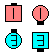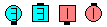Reach Out\$B\$NA0(B \$B8e(B

Tandem Couples \$B\$+\$i(B: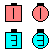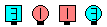Reach Out\$B\$NA0(B \$B8e(B

T-Bone 2 x 2 \$B\$+\$i(B: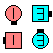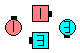Reach Out\$B\$NA0(B \$B8e(B

\$BCm

• Trailers \$B\$OIaDL\$*8_\$\$\$K>/\$7lightly tap \$B\$O(B, \$B\$O\$?\$/\$H\$\$\$&\$h\$j\$U\$l\$F\$G\$7\$g\$&\$+(B) \$B\$+\$i(B, \$B30\$X(B flip \$B\$7\$^\$9!%(B
• \$B%*%j%8%J%k\$N(B Leaders \$B\$O(B Roll \$B\$G\$-\$^\$;\$s!%(B
• \$B1&\$N(B Mini-Wave \$B\$N(B Box \$B\$+\$i(B, Reach Out \$B\$O(B Turn To A Line [C2] \$B\$HF1\$8F0\$-\$K\$J\$j\$^\$9!%(B
• Cross Reach Out [NOL]: Trailers \$B\$O(B 1/2 Box Circulate \$B\$r\$7\$F(B, Phantom Cross Run (Trade and Spread) \$B\$r\$7(B, Leaders \$B\$O(B Box Circulate 1 & 1/2 \$B\$r\$7\$^\$9!%(BScoot Apart (\$B2<\$KDj5A\$,\$"\$j\$^\$9(B) \$B\$HF1\$87k2L\$K\$J\$j\$^\$9!%(B

Scoot Apart [C4] (Jim Davis 1977): Mini-Wave \$B\$N(B Box \$B\$^\$?\$OE,@Z\$J(B 2 x 2 \$B\$N(B T-Bone \$B\$+\$i!%(B Trailers \$B\$O(B Extend, Trade & Spread \$B\$r\$7(B, Leaders \$B\$O(B Box Circulate 1 & 1/2 \$B\$r\$7\$^\$9!%(BMini-Wave \$B\$N(B Box \$B\$+\$i\$O(B, Two-Faced Line \$B\$G=*\$o\$j\$^\$9!%(B

Cross Scoot Apart [C4] (Jim Davis 1977): Trailers \$B\$O(B Cross Extend, Trade & Spread \$B\$r\$7(B, Leaders \$B\$O(B Box Circulate 1 & 1/2 \$B\$r\$7\$^\$9!%(BMini-Wave \$B\$N(B Box \$B\$+\$i\$O(B, \$BH?BP\$NCriss Cross Scoot Apart [C4]: Trailers \$B\$O(B Cross Extend, Trade & Spread \$B\$r\$7(B, Leaders \$B\$O(B Box Circulate & Cross Extend \$B\$r\$7\$^\$9!%(BMini-Wave \$B\$N(B Box \$B\$+\$i\$O(B, \$BH?BP\$NCALLERLAB definition for Reach OutChoreography for Reach OutComments? Questions? Suggestions?

https://www.ceder.net/def/reachout.php?level=C3A&language=japan
06-December-2019 04:17:27